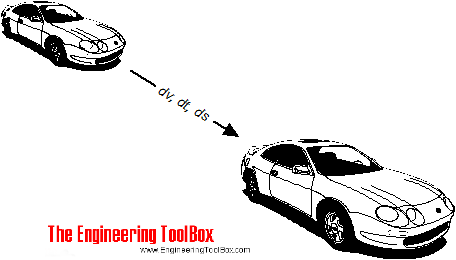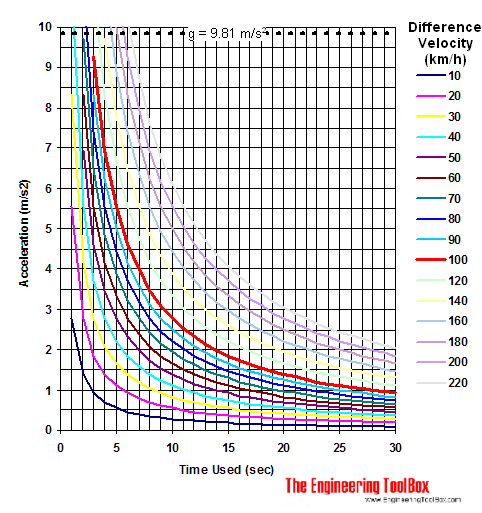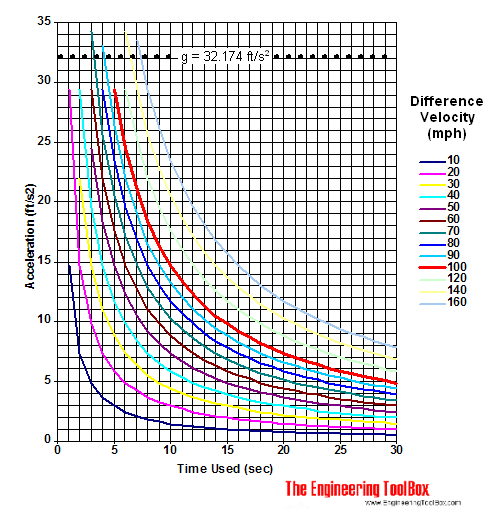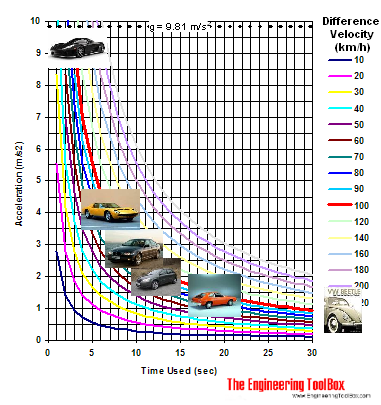Engineering ToolBox - Resources, Tools and Basic Information for Engineering and Design of Technical Applications!

# Car Acceleration

## Car acceleration calculator.If you know the initial and final velocity of a car (or whatever) - and the time used - the average acceleration can be calculated as

a = dv / dt

= (vf - vs) / dt                                           (1)

where

a = acceleration of object (m/s2, ft/s2)

dv = change in velocity (m/s, ft/s)

vf = final speed (m/s, ft/s)

vs = start speed (m/s, ft/s)

dt = time used (s)

Common benchmark velocities for acceleration of cars and motorcycles are

• 0 - 60 mph = 0 - 26.8 m/s = 0 - 96.6 km/h
• 0 - 100 km/h = 0 - 27.8 m/s = 0 - 62.1 mph

### Online Car Acceleration Calculator

km/h

start speed (km/h)

final speed (km/h)

time used (s)

mass of object (kg)

Note that force, work and power are calculated for mass acceleration only. Forces due to air resistance (drag) and rolling friction are not included.

#### mph

start speed (mph)

final speed (mph)

time used (s)

### Car Acceleration Diagram - km/h### Car Acceleration Diagram - mphIf you know the distance moved and the time used - the acceleration can be calculated as

a = 2 ds / dt2                                 (2)

where

ds = distance moved (m, ft)

### Acceleration of some known cars### Acceleration Force

The acceleration force can be calculated as

F = m a                                (3)

where

F = acceleration force (N, lbf)

m = mass of car (kg, slugs)

### Acceleration Work

The acceleration work can be calculated as

W = F l                                    (4)

where

W = work done (Nm, J, ft lbf)

l = distance moved (m, ft)

### Acceleration Power

The acceleration power can be calculated as

P = W / dt                                  (5)

where

P = power (J/s, W, ft lbf/s)

### Example - Car Acceleration

A car with mass 1000 kg (2205 lbm) accelerates from 0 m/s (0 ft/s) to 27.8 m/s (100 km/h, 91.1 ft/s, 62.1 mph) in 10 s.

The acceleration can be calculated from eq. 1 as

a = ((27.8 m/s) - (0 m/s)) / (10 s)

= 2.78 m/s2

The acceleration force can be calculated from eq. 3 as

F = (1000 kg) (2.78 m/s2)

= 2780 N

The distance moved can be calculated by rearranging eq. 2 to

ds = a dt2 / 2

= (2.78 m/s2) (10 s)2 / 2

= 139 m

The acceleration work can be calculated from eq. 4 as

W = (2780 N) (139 m)

= 386420 J

The acceleration power can be calculated from eq. 5 as

P = (386420 J) / (10 s)

= 38642 W

= 38.6 kW

The calculation can also be done in Imperial units:

The acceleration can be calculated from eq. 1 as

a = ((91.1 ft/s) - (0 ft/s)) / (10 s)

= 9.11 ft/s2

In the Imperial system mass is measured in slugs where 1 slug = 32.17405 lbm

The acceleration force can be calculated from eq. 3 as

F = ((2205 lbm) (1/32.17405 (slugs/ lbm)) ) (9.11 ft/s2)

= 624 lbf

The distance moved can be calculated by rearranging eq. 2 to

ds = a dt2 / 2

= (9.11 ft/s2) (10 s)2 / 2

= 455 ft

The acceleration work can be calculated from eq. 4 as

W = (624 lbf) (455 ft)

= 284075 ft lbf

• 1 ft lbf = 1.36 J

The acceleration power can be calculated from eq. 5 as

P = (284075 ft lbf) / (10 s)

= 28407 ft lbf/s

• 1 ft lbf/s = 1.36 W = 0.00182 hp

## Related Topics

• Dynamics - Motion - velocity and acceleration, forces and torque.
• Mechanics - Forces, acceleration, displacement, vectors, motion, momentum, energy of objects and more.

## Engineering ToolBox - SketchUp Extension - Online 3D modeling!

Add standard and customized parametric components - like flange beams, lumbers, piping, stairs and more - to your Sketchup model with the Engineering ToolBox - SketchUp Extension - enabled for use with the amazing, fun and free SketchUp Make and SketchUp Pro .Add the Engineering ToolBox extension to your SketchUp from the SketchUp Pro Sketchup Extension Warehouse!

Translate

## Privacy

We don't collect information from our users. Only emails and answers are saved in our archive. Cookies are only used in the browser to improve user experience.

Some of our calculators and applications let you save application data to your local computer. These applications will - due to browser restrictions - send data between your browser and our server. We don't save this data.

## Citation

• Engineering ToolBox, (2008). Car Acceleration. [online] Available at: https://www.engineeringtoolbox.com/car-acceleration-d_1309.html [Accessed Day Mo. Year].

Modify access date.

. .

#### Scientific Online Calculator6 24